# Land Area Calculator or Measurement Methods: Planimeter, Geometric, Measuring Wheel Methods

Page content

## Methods of Calculating or Measuring the Area of Land

Land needs to be measured for various reasons like prior to purchase, when doing stock taking, building a boundary wall, dispute with a neighbor over demarcation, etc. Measuring regular land area like residential plots sold by real estate agents and land developers is easy as they have mostly rectangle or other geometrical shapes. Recalling geometry studied in High School we can do the measurements and arrive at the results. But if the area of land is highly irregularly shaped or if you are purchasing large tracts of land like a farm measuring miles across, then how do you do the measurements?

You can always make use of the services of a land surveyor or a civil engineer, but that is expensive. From time to time you want to measure the area of your land and calculate how much it is worth. In this article we explain some do-it-yourself methods of measuring your land and some high technology solutions.

We will be discussing the measuring of land using simple geometry, using maps and planimeter, GPS, Aerial photography, Satellite imagery, using Google earth, using maps and graphs, and by using measuring wheels in this article.

## Geometric Method

In this method the odd shaped plot is surveyed and plotted over graph paper. The area of the plot is then subdivided into geometric figures whose area we can calculate by formulae.

First the largest size geomatric figure that can be neatly be drawn in the map is made, followed by smaller shapes, until the complete space has been occupied more or less.

All these figures have their areas calculated, which are then added together to give the total area.

For example:

Area of Rectangle: Length x Breadth

Area of Square: Length x Length

Area of Triangle: 0.5 x Base x Height

Area of Trapezium: 0.5 x (Length 1 + Length 2) x Height

By the use of these formulas the correct area can be arrived at.

## Using Planimeter on Map

A small device called planimeter is used for measuring areas of a graphically represented planar region. The planimeter can measure all regular and irregular shapes. All you have to do is to pivot it at any point and then run the end pin all over the perimeter of the area you want to measure. It has got a flexible linkage which allows it to move without any effort in all directions.

But using a planimeter is only useful if you have a map of the area or an aerial photograph. Nowadays you can get high resolution photos by satellites by using Google earth, wikimapia, and other applications. You can also draw your own map after you have taken the dimensions and angles of the various lines and afterwards measure it using a planimeter.

One problem that can occur is that of scale. The planimeter will give the area measurement in its own scale or square meter and you have to convert it to your own unit. By having a known area at hand on the map or by drawing a small square within the map you can calculate its area as you know the scale ratio of the map. Then you run the planimeter over it and get its evaluation.

For example if your square is 300 square feet and the planimeter measures it as 1.5 square meter then

1 m2= 200 feet square

Thus multiplying the final reading of the planimeter in m2 by 2 will give you the reading in square feet.

## Planimeter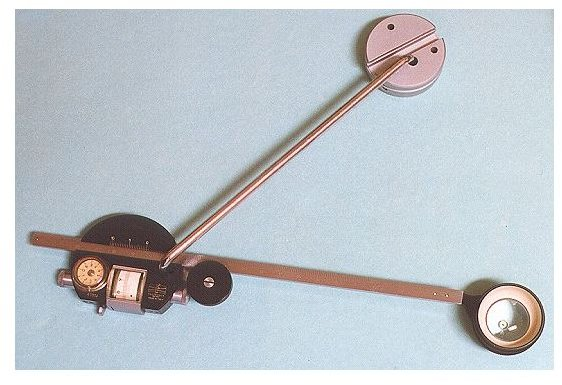## By Using Measuring Wheel

A measuring wheel is another method of measuring the area. In principle it is like a planimeter that you have to go over the perimeter of the area with it, only you have to do it on the actual land. Built like a single bicycle wheel, it has to be run all over the edges of the land to be measured and it gives the reading in units chosen when you reach the starting point.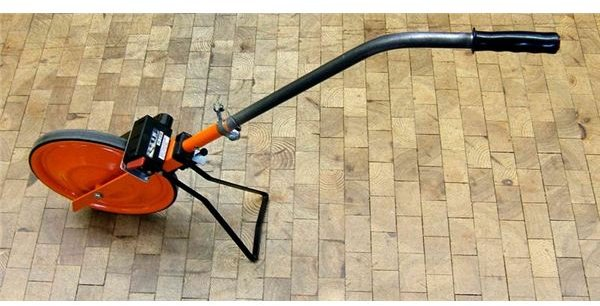## By using Graph Paper

In this method the map is superimposed on a translucent sheet or you can take a Photostat on a transparent slide. Then it can be put over a suitable sized graph paper and clamped together. As the area of each square of the graph is known, measuring the approximate number of squares and multiplying it by the area and the scale will give you the area. In this method the aerial photos or maps can be used.

## Land Marked on Graph Paper for Calculation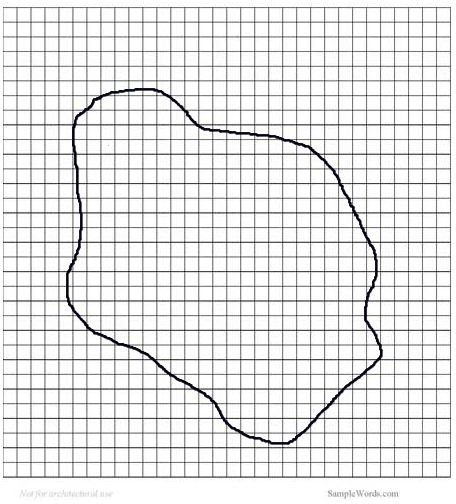## Using GPS

GPS stands for global positioning system and consists of sets of satellites that give your coordinates when you use a hand held receiver. It gives your position coordinates with an accuracy of within 1 meter. This is very useful in large land areas where you can go to each end and note the coordinates and later calculate the area.

## GPS Reciever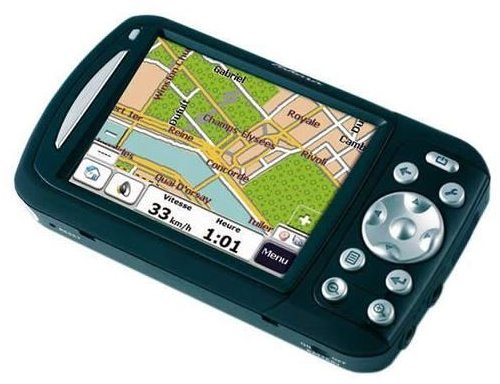## Using Aerial Photographs

The aerial photographs of the land areas can also be used for the measurement by using a planimeter or by graph paper using suitable scale. The aerial photographs are very useful in land surveys. This is very useful if the land has visible markings or can be seen properly or having contrast. Like in the figure given below the farmed lands can be differentiated from the non farmed land areas.

## Aerial Photo of Farm in US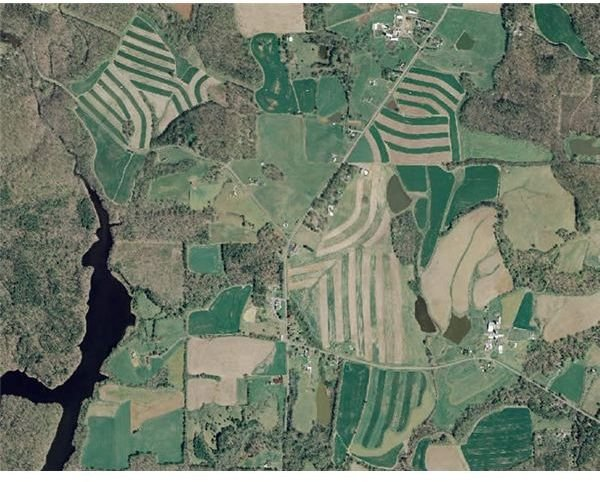## Image Credits

1. Measuring Wheel
2. Planimeter:
3. Division into Geometric Shapes: https://whistleralley.com/planimeter/tile1.gif
4. Aerial Photo of Farm: https://www.co.orange.nc.us/soilwater/images/AerialPhoto_Farm.jpg
5. GPS Reciever: https://www.navigadget.com/wp-content/postimages/2007/07/takara-gp4-gps-01.jpg